# 打假：印度是强奸之国

## 均值分析

globalmean <- rape %>%
group_by(Year) %>%
summarise(mean=round(mean(Events,na.rm=T),2),
median=median(Events,na.rm=T),
sd=round(sd(Events,na.rm=T),2))

datatable(globalmean,options = list(pageLength=20))

p <- ggplot(globalmean,aes(Year,mean,fill=sd))+geom_bar(stat="identity")+
scale_fill_distiller(palette = "BrBG") +
ylab("Mean Value")
p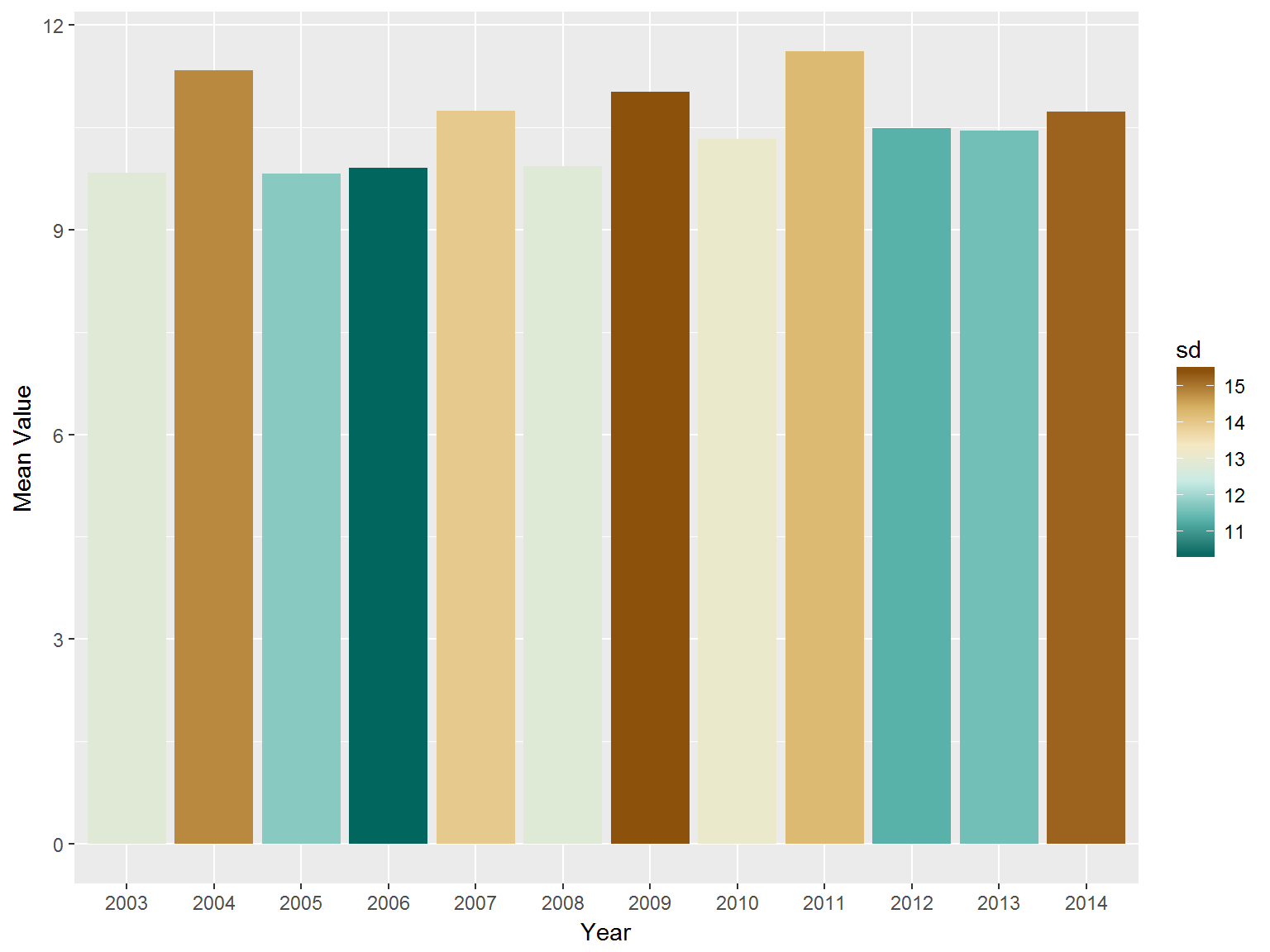## 分布分析

p <- ggplot(rape,aes(Year,Events,fill=Year)) +
theme(legend.position = "none") +
scale_fill_brewer(palette = "Paired")

ggplotly(p + geom_boxplot(na.rm = T))boxplot不能看出分布，所以我们使用violinplot来查看一下每年的分布情况：

p2 <- p + geom_violin(na.rm = T)
ggplotly(p2)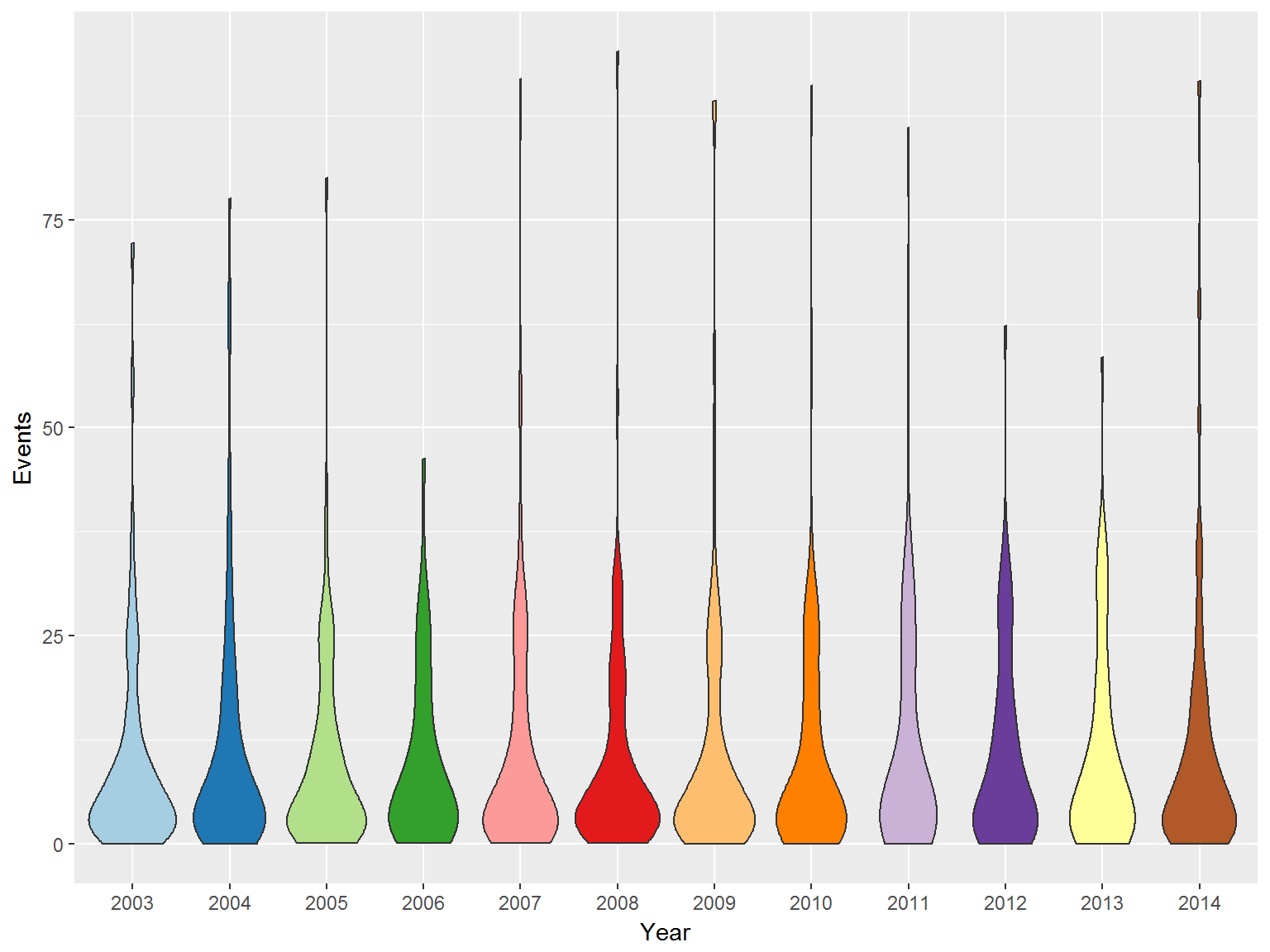## 印度在分布图中的位置

pindia <- p2 +
geom_point(data = rape %>% filter(Country=="India"),shape=3,size=5,color="darkblue") + scale_fill_brewer(palette = "Paired")
## Scale for 'fill' is already present. Adding another scale for 'fill',
## which will replace the existing scale.
ggplotly(pindia)
## Warning: Removed 2 rows containing missing values (geom_point).pindia +  ylim(0,10)
## Warning: Removed 2 rows containing missing values (geom_point).## 各大洲的情况

p <- ggplot(rape,aes(Region, Events, fill = Region)) +
geom_violin(na.rm=T) +
scale_fill_brewer(palette = "Paired")
ggplotly(p)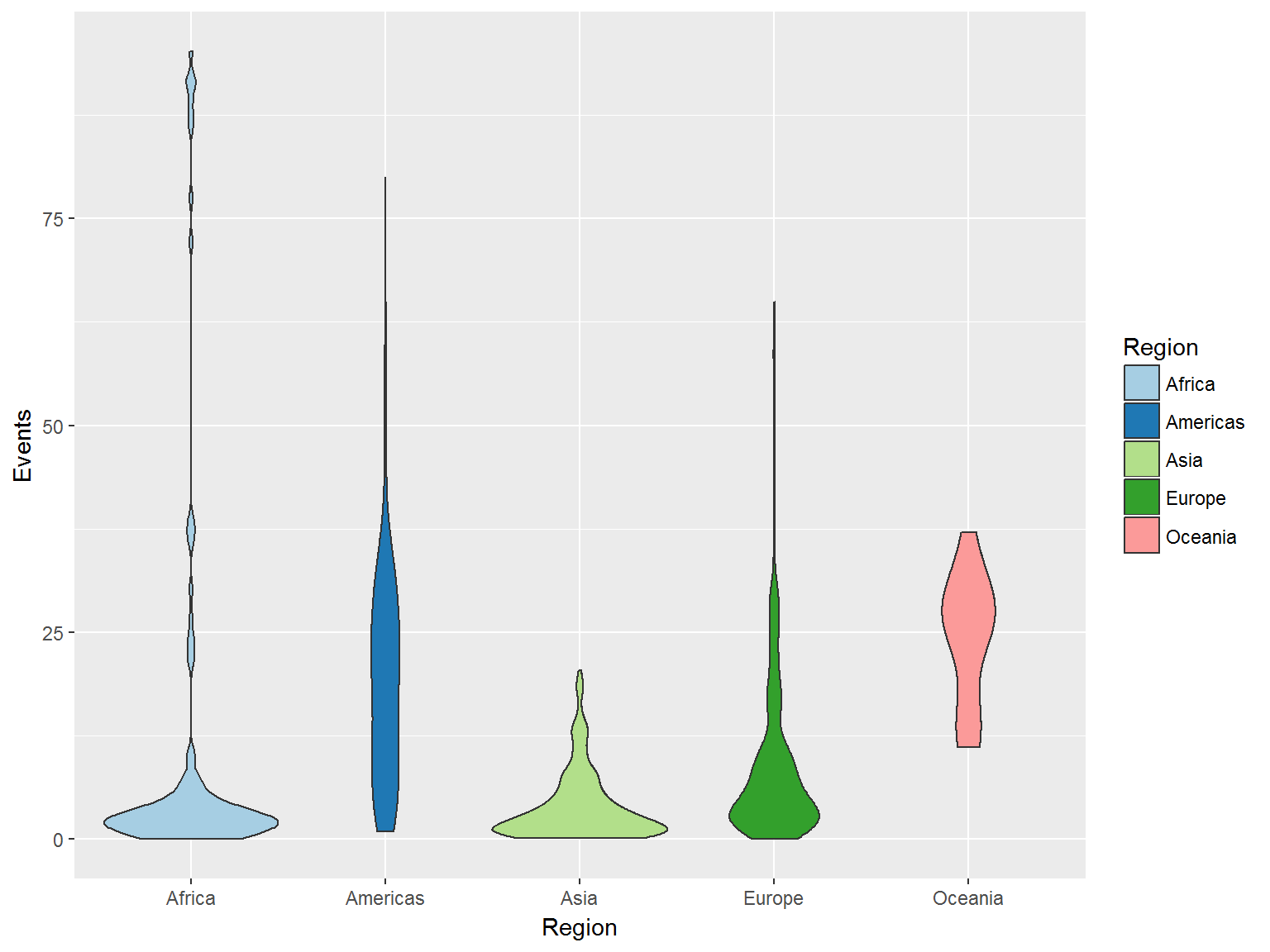globalmean <- rape %>%
group_by(Region) %>%
summarise(mean=round(mean(Events,na.rm=T),2),
median=median(Events,na.rm=T),
sd=round(sd(Events,na.rm=T),2))

knitr::kable(globalmean)
Region mean median sd
Africa 10.66 2.60 22.43
Americas 19.30 18.80 12.74
Asia 3.92 2.50 4.15
Europe 9.33 5.60 10.54
Oceania 24.31 26.25 7.87

orape <- rape
rape$Country <- unlist(lapply(rape$Country, function(x) substr(x,1,10)))

ggplotly(ggplot(rape,aes(Sub Region, Events, fill = Sub Region)) +
theme(legend.position = "none",
axis.text.x = element_text(vjust = 0.25,angle = 90)) +
geom_violin(na.rm=T) )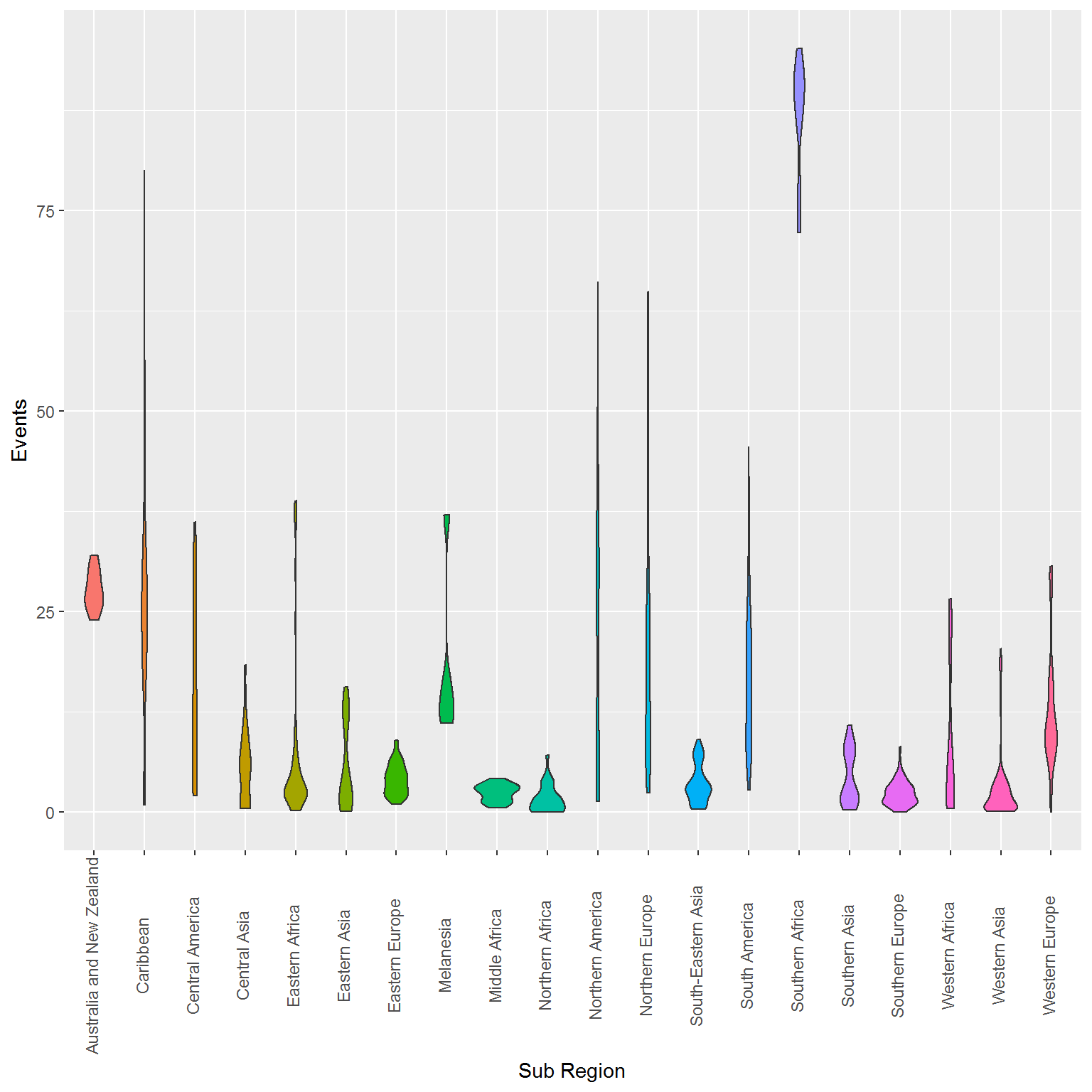## 亚洲地区的数据

1. 作为发达国家，日本的罪案率很低。
2. 印度在亚洲国家中也算罪案率不高的。也就是考虑到亚洲地区记录在案的犯罪比例可能偏低，那么在同一地区而言，印度的记录也不算很高。比如附近的泰国罪案率就远高于印度。虽然对于印度及其周边国家的文化并不了解，不过认为印度的罪案记录率又远低于周边地区的话似乎也不是很有道理的假设。
3. 邻国蒙古亮了。
p <- ggplot(rape %>%
filter(Region=="Asia"),
aes(Country, Events, fill = Country)) +
theme(legend.position = "none",
axis.text.x = element_text(vjust = 0.25,angle = 90)) +
geom_violin(na.rm=T)
ggplotly(p)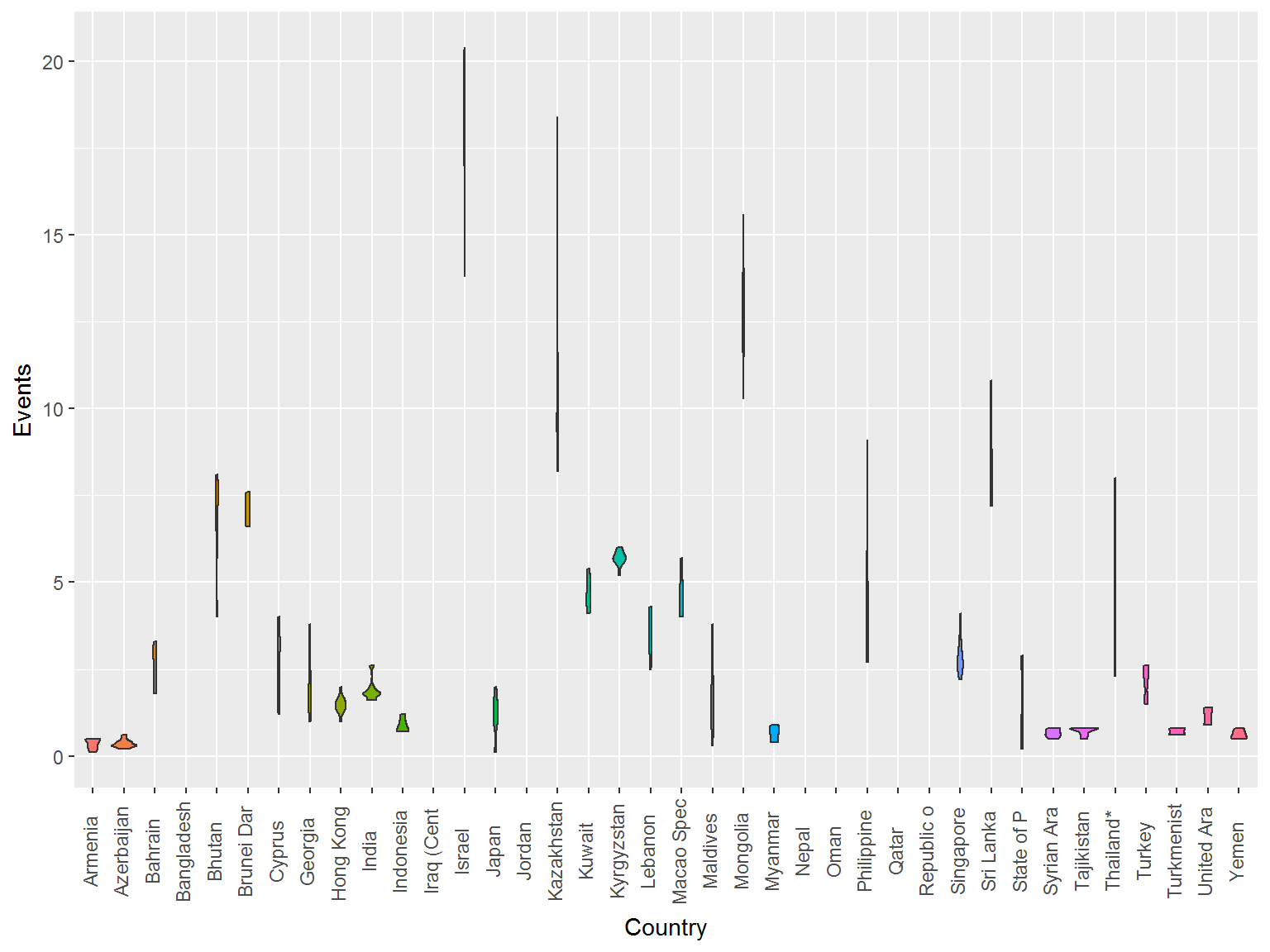## 欧洲地区的数据

p <- ggplot(rape %>%
filter(Region=="Europe"),
aes(Country, Events, fill = Country)) +
theme(legend.position = "none",
axis.text.x = element_text(vjust = 0.25,angle = 90)) +
geom_violin(na.rm=T)
ggplotly(p)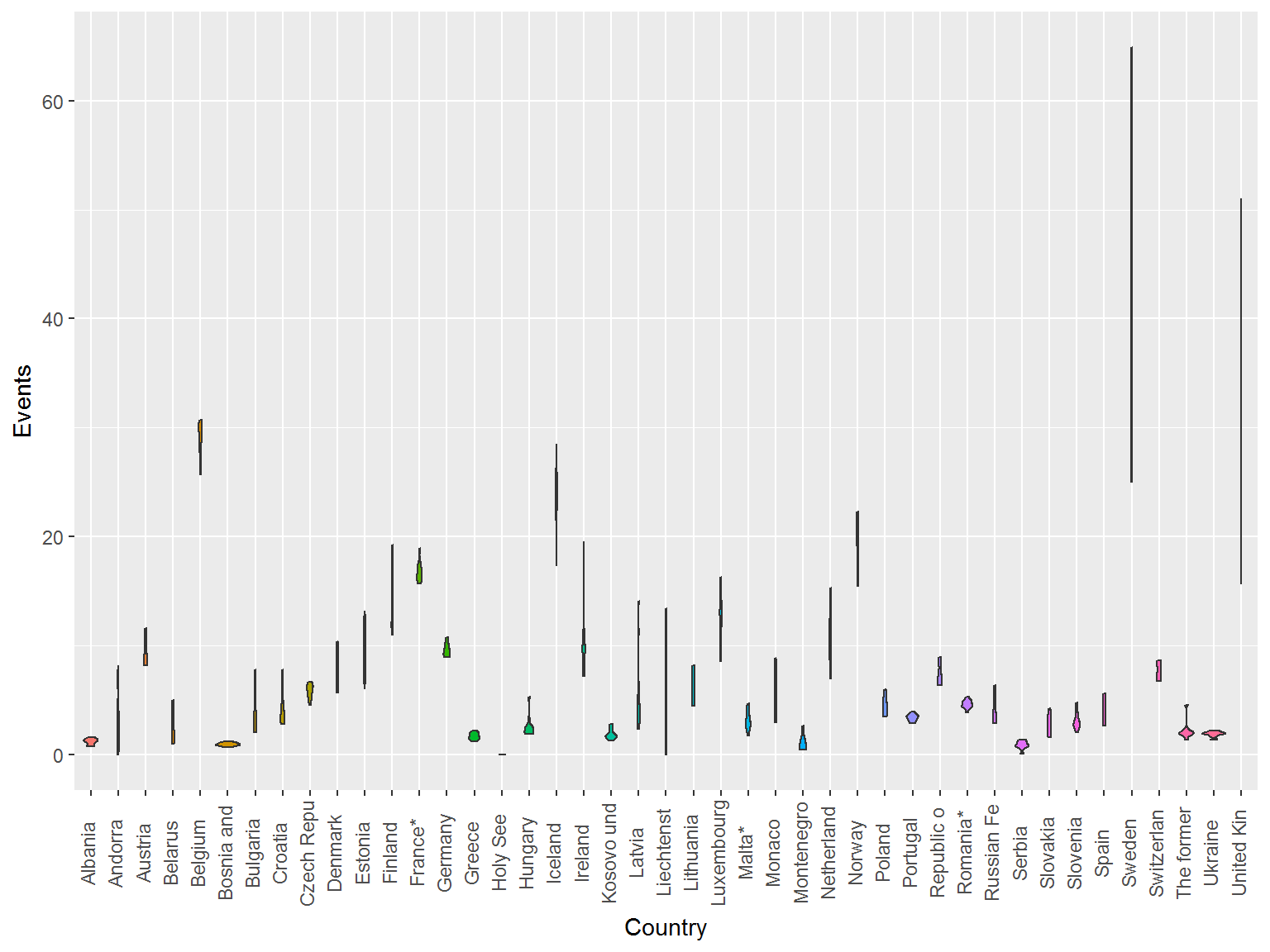p <- ggplot(rape %>%
filter(Sub Region=="Northern Europe"),
aes(Country, Events, fill = Country)) +
theme(legend.position = "none",
axis.text.x = element_text(vjust = 0.25,angle = 90)) +
geom_violin(na.rm=T)
ggplotly(p)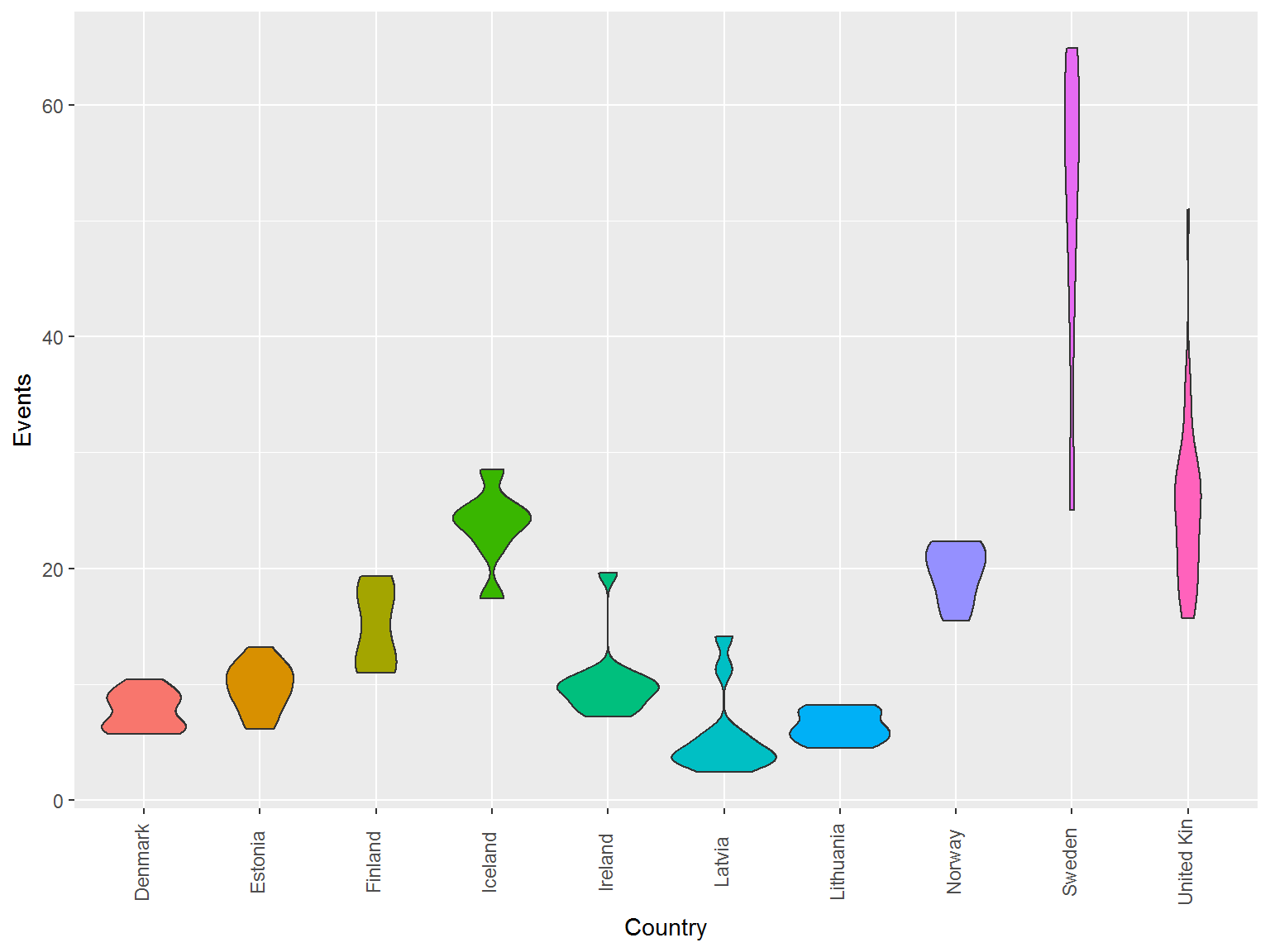## 各国的数据

datatable(orape %>% group_by(Country) %>%
summarise(Mean=round(mean(Events,na.rm=T),2),
Median=round(median(Events,na.rm=T),2),
SD=round(sd(Events,na.rm=T),2)) %>%
arrange(desc(Mean)),filter="top",options = list(pageLength=25))

## 展开本分类索引